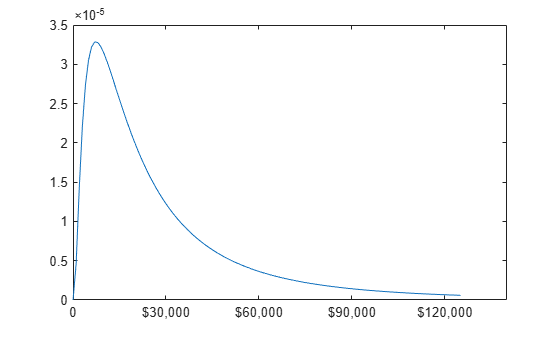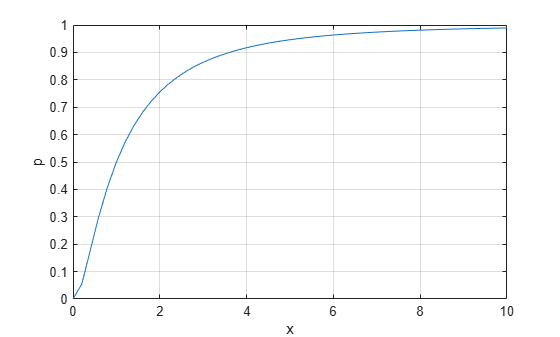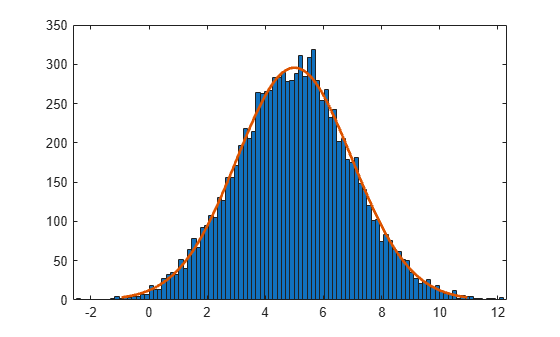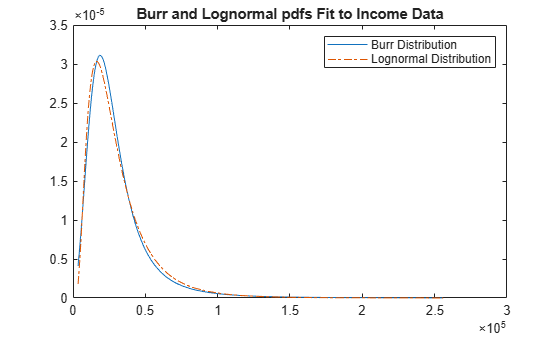## Lognormal Distribution

### Overview

The lognormal distribution, sometimes called the Galton distribution, is a probability distribution whose logarithm has a normal distribution. The lognormal distribution is applicable when the quantity of interest must be positive, because log(x) exists only when x is positive.

Statistics and Machine Learning Toolbox™ offers several ways to work with the lognormal distribution.

### Parameters

The lognormal distribution uses these parameters.

ParameterDescriptionSupport
`mu` (μ)Mean of logarithmic values$-\infty <\mu <\infty$
`sigma` (σ)Standard deviation of logarithmic values$\sigma \ge 0$

If X follows the lognormal distribution with parameters µ and σ, then log(X) follows the normal distribution with mean µ and standard deviation σ.

#### Parameter Estimation

To fit the lognormal distribution to data and find the parameter estimates, use `lognfit`, `fitdist`, or `mle`.

• For uncensored data, `lognfit` and `fitdist` find the unbiased estimates of the distribution parameters, and `mle` finds the maximum likelihood estimates.

• For censored data, `lognfit`, `fitdist`, and `mle` find the maximum likelihood estimates.

Unlike `lognfit` and `mle`, which return parameter estimates, `fitdist` returns the fitted probability distribution object `LognormalDistribution`. The object properties `mu` and `sigma` store the parameter estimates.

#### Descriptive Statistics

The mean m and variance v of a lognormal random variable are functions of the lognormal distribution parameters µ and σ:

`$\begin{array}{l}m=\mathrm{exp}\left(\mu +{\sigma }^{2}/2\right)\\ v=\mathrm{exp}\left(2\mu +{\sigma }^{2}\right)\left(\mathrm{exp}\left({\sigma }^{2}\right)-1\right)\end{array}$`

Also, you can compute the lognormal distribution parameters µ and σ from the mean m and variance v:

`$\begin{array}{l}\mu =\mathrm{log}\left({m}^{2}/\sqrt{v+{m}^{2}}\right)\\ \sigma =\sqrt{\mathrm{log}\left(v/{m}^{2}+1\right)}\end{array}$`

### Probability Density Function

The probability density function (pdf) of the lognormal distribution is

`$y=f\left(x|\mu ,\sigma \right)=\frac{1}{x\sigma \sqrt{2\pi }}\mathrm{exp}\left\{\frac{-{\left(\mathrm{log}x-\mu \right)}^{2}}{2{\sigma }^{2}}\right\},\text{ }\text{for}\text{\hspace{0.17em}}x>0.$`

For an example, see Compute Lognormal Distribution pdf.

### Cumulative Distribution Function

The cumulative distribution function (cdf) of the lognormal distribution is

`$p=F\left(x|\mu ,\sigma \right)=\frac{1}{\sigma \sqrt{2\pi }}{\int }_{0}^{x}\frac{1}{t}\mathrm{exp}\left\{\frac{-{\left(\mathrm{log}t-\mu \right)}^{2}}{2{\sigma }^{2}}\right\}dt,\text{ }\text{for}\text{\hspace{0.17em}}x>0.$`

For an example, see Compute Lognormal Distribution cdf.

### Examples

#### Compute Lognormal Distribution pdf

Suppose the income of a family of four in the United States follows a lognormal distribution with `mu = log(20,000)` and `sigma = 1`. Compute and plot the income density.

Create a lognormal distribution object by specifying the parameter values.

`pd = makedist('Lognormal','mu',log(20000),'sigma',1)`
```pd = LognormalDistribution Lognormal distribution mu = 9.90349 sigma = 1 ```

Compute the pdf values.

```x = (10:1000:125010)'; y = pdf(pd,x);```

Plot the pdf.

```plot(x,y) h = gca; h.XTick = [0 30000 60000 90000 120000]; h.XTickLabel = {'0','\$30,000','\$60,000',... '\$90,000','\$120,000'};```#### Compute Lognormal Distribution cdf

Compute the cdf values evaluated at the values in `x` for the lognormal distribution with mean `mu` and standard deviation `sigma`.

```x = 0:0.2:10; mu = 0; sigma = 1; p = logncdf(x,mu,sigma);```

Plot the cdf.

```plot(x,p) grid on xlabel('x') ylabel('p')```#### Relationship Between Normal and Lognormal Distributions

If X follows the lognormal distribution with parameters µ and σ, then log(X) follows the normal distribution with mean µ and standard deviation σ. Use distribution objects to inspect the relationship between normal and lognormal distributions.

Create a lognormal distribution object by specifying the parameter values.

`pd = makedist('Lognormal','mu',5,'sigma',2)`
```pd = LognormalDistribution Lognormal distribution mu = 5 sigma = 2 ```

Compute the mean of the lognormal distribution.

`mean(pd)`
```ans = 1.0966e+03 ```

The mean of the lognormal distribution is not equal to the `mu` parameter. The mean of the logarithmic values is equal to `mu`. Confirm this relationship by generating random numbers.

Generate random numbers from the lognormal distribution and compute their log values.

```rng('default'); % For reproducibility x = random(pd,10000,1); logx = log(x);```

Compute the mean of the logarithmic values.

`m = mean(logx)`
```m = 5.0033 ```

The mean of the log of `x` is close to the `mu` parameter of `x`, because `x` has a lognormal distribution.

Construct a histogram of `logx` with a normal distribution fit.

`histfit(logx)`The plot shows that the log values of `x` are normally distributed.

`histfit` uses `fitdist` to fit a distribution to data. Use `fitdist` to obtain parameters used in fitting.

`pd_normal = fitdist(logx,'Normal')`
```pd_normal = NormalDistribution Normal distribution mu = 5.00332 [4.96445, 5.04219] sigma = 1.98296 [1.95585, 2.01083] ```

The estimated normal distribution parameters are close to the lognormal distribution parameters 5 and 2.

#### Compare Lognormal and Burr Distribution pdfs

Compare the lognormal pdf to the Burr pdf using income data generated from a lognormal distribution.

Generate the income data.

```rng('default') % For reproducibility y = random('Lognormal',log(25000),0.65,[500,1]);```

Fit a Burr distribution.

`pd = fitdist(y,'burr')`
```pd = BurrDistribution Burr distribution alpha = 26007.2 [21165.5, 31956.4] c = 2.63743 [2.3053, 3.0174] k = 1.09658 [0.775479, 1.55064] ```

Plot both the Burr and lognormal pdfs of income data on the same figure.

```p_burr = pdf(pd,sortrows(y)); p_lognormal = pdf('Lognormal',sortrows(y),log(25000),0.65); plot(sortrows(y),p_burr,'-',sortrows(y),p_lognormal,'-.') title('Burr and Lognormal pdfs Fit to Income Data') legend('Burr Distribution','Lognormal Distribution')```### Related Distributions

 Abramowitz, Milton, and Irene A. Stegun, eds. Handbook of Mathematical Functions: With Formulas, Graphs, and Mathematical Tables. 9. Dover print.; [Nachdr. der Ausg. von 1972]. Dover Books on Mathematics. New York, NY: Dover Publ, 2013.

 Evans, M., N. Hastings, and B. Peacock. Statistical Distributions. 2nd ed., Hoboken, NJ: John Wiley & Sons, Inc., 1993.

 Lawless, J. F. Statistical Models and Methods for Lifetime Data. Hoboken, NJ: Wiley-Interscience, 1982.

 Marsaglia, G., and W. W. Tsang. “A Fast, Easily Implemented Method for Sampling from Decreasing or Symmetric Unimodal Density Functions.” SIAM Journal on Scientific and Statistical Computing. Vol. 5, Number 2, 1984, pp. 349–359.

 Meeker, W. Q., and L. A. Escobar. Statistical Methods for Reliability Data. Hoboken, NJ: John Wiley & Sons, Inc., 1998.

 Mood, A. M., F. A. Graybill, and D. C. Boes. Introduction to the Theory of Statistics. 3rd ed., New York: McGraw-Hill, 1974. pp. 540–541.

﻿